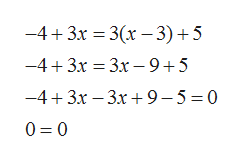Question
1 views

For Exercise, solve the equation.

−4 + 3x= 3(x − 3) + 5

check_circle

Step 1

Given equation is

Step 2

On solvin...help_outlineImage Transcriptionclose-4+3x = 3(x – 3)+5 -4+ 3x = 3x – 9+5 -4+3x – 3x+9-5 =0 0 = 0 fullscreen

### Want to see the full answer?

See Solution

#### Want to see this answer and more?

Solutions are written by subject experts who are available 24/7. Questions are typically answered within 1 hour.*

See Solution
*Response times may vary by subject and question.
Tagged in

### Equations and In-equations